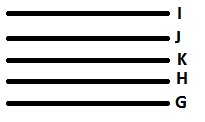All the roads of a city are either perpendicular or parallel to one another. The roads are all straight. Roads A, B, C, D and E are parallel to one another. Roads G, H, I, J, K, L and M are parallel to one another.

If K is parallel to I and K is ½ km south of J and 1 km north of G. which two roads would be ½ km apart ?

###### Option:
A. I and K
B. J and G
C. I and G
D. J and H
E. K and J
Answer: E . K and J

Justification:

Clearly from statements (1) and (2), figure 1 follows; from statement (3), figure 2 follows; from statement (4), figure 3 follows; and from statement (5), figure 4 follows; and from statements (6) and (7), figure 5 follows.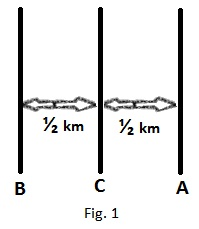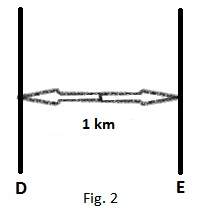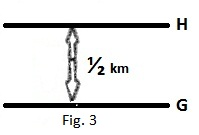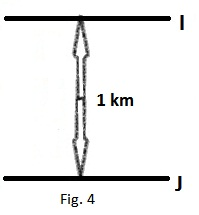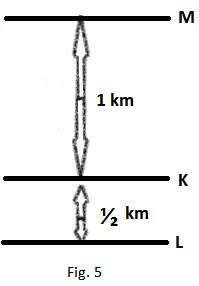Clearly as per the information combination with figures 3 and 4, the figure is as shown. The two roads J and K, K and H &  H and G ½ km apart, So, the answer is K and J.